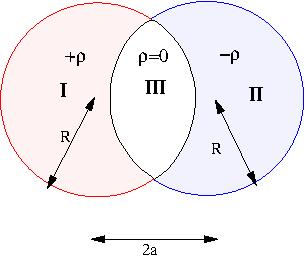# [YMP/EM-02011] Overlapping Spheres -II

For page specific messages
For page author info
 ProblemTwo spheres each of radius $R$ are placed so that they partially overlap. The charge densities in the overlap region is zero and in the two non overlapping regions is $+\rho$ and $-\rho$ respectively as shown in figure. The separation between the centers of the spheres is $D$.Find the electric field in the two non-overlap regions.Solution
Let the centres of the two spheres be at $\vec{r}_1$ be the position vector of a point in the overlap region drawn from the
center of the first sphere and $\vec{r}_2$ be the position vector of the same point from the center of the second sphere.
The given charge density is equivalent to the two spheres having charge densities $$\pm\rho$$  throughout their respective
volumes. Thus the result for a uniformly charged sphere can be applied to the individual spheres

Using superposition principle

• the electric field in the overlap region due to the first sphere =  $\displaystyle \frac{\rho \vec{r_1}}{3\varepsilon_0}$
• the electric field due to the second sphere = $\displaystyle - \frac{\rho \vec{r_2}}{3\varepsilon_0}$

Thus the total electric field, using superposition principle,
$$\vec {E}= \frac{\rho(\vec{r}_1-\vec{r}_2)}{3\varepsilon_0} = \frac{\rho \vec{D}}{3\varepsilon_0}$$
where   $\vec{D}=\vec{r}_1-\vec{r_2}$, is the
separation between the centres of the two spheres.
Thus the electric field is constant.

n
0

X